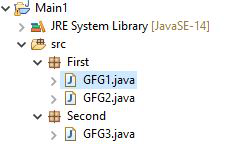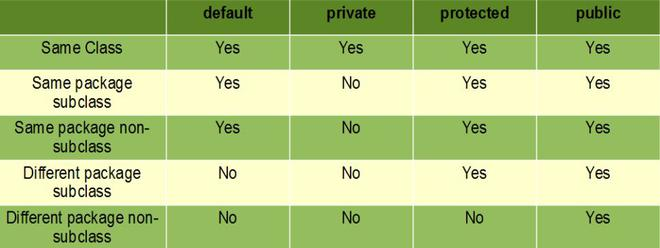# How to Import Custom Class in Java?

• Difficulty Level : Expert
• Last Updated : 10 Oct, 2021

Java language is one of the most popular languages among all programming languages. There are several advantages of using the java programming language, whether for security purposes or building large distribution projects. One of the advantages of using Java is that it tries to connect every concept in the language to the real world with the help of the concepts of classes, inheritance, polymorphism, etc. In this article, we will discuss how to import custom classes from one project to another project or in the same project.

Custom classes are created by the user for a particular purpose. These classes are not present in the system library of the project. Consider the structure of the project depicted below as follows:

Attention reader! Don’t stop learning now. Get hold of all the important Java Foundation and Collections concepts with the Fundamentals of Java and Java Collections Course at a student-friendly price and become industry ready. To complete your preparation from learning a language to DS Algo and many more,  please refer Complete Interview Preparation Course.Structure Format:

```ProjectName:Main1
|
|
First(package)[ GFG1.java , GFG2.java ] (classes)
|
Second(package)[GFG3.java] (class)```

Note: In order to get through the concept, one must go through access modifiers in java to understand the concept of the scope of classes, members, and methods.Here we will be going through both the structure types as follows which later on will be implementing the same further below:

1. Custom class within the same package
2. Custom class from another package

Implementation: Importing classes for the same project

Classes in the same project can be imported into any other class in the same project without any import statement in the particular class of the project. Because, by default, all the class members and methods are of default modifier and, according to the scope of the default modifier, they can be accessed in the same package / same package subclass without importing the class. Refer to the above table.

Example 1: GFG1.java

## Java

 `// Package of the class``package` `First;` `public` `class` `GFG1 {` `    ``int` `a;``    ``int` `b;``    ``// Creating constructor``    ``GFG1(``int` `a, ``int` `b)``    ``{``        ``this``.a = a;``        ``this``.b = b;``    ``}``    ``// Function to add the members of the class``    ``int` `add() { ``return` `this``.a + ``this``.b; }``}`

Example 2: GFG2.java

## Java

 `// Package first``package` `First;` `public` `class` `GFG2 {` `    ``public` `static` `void` `main(String[] args)``    ``{``        ``// Creating of instance of second class present``        ``// in the same project``        ``GFG1 ob = ``new` `GFG1(``1``, ``2``);``        ``System.out.println(``"Addition "` `+ ob.add());``    ``}``}`

Output:

`Addition 3`

Let us do dwell on next where we will be importing custom class from another package.

Implementation:

Classes are imported between the different packages only when particular that need to be imported are marked as public and its member and methods should be of public so that they can be used outside its package.

Example 1: Second package class

## Java

 `package` `Second;` `class` `GFG3 {``    ``int` `a;``    ``int` `b;``    ``// Creating constructor``    ``public` `GFG3(``int` `a, ``int` `b)``    ``{``        ``this``.a = a;``        ``this``.b = b;``    ``} ``// Function to subtract the values``    ``public` `int` `subtract() { ``return` `this``.a - ``this``.b }``}`
```Above second package class is imported to the class of first package
Structure:
Second:(Package)
|
GFG3
First:(Package)
|
GFG2```

Example 2: First package class

## Java

 `package` `First;``// Importing class of second package``import` `Second.GFG3;` `public` `class` `GFG2 {` `    ``public` `static` `void` `main(String[] args)``    ``{``        ``// Creating reference of the GFG1 class that is``        ``// present in the same project``        ``GFG1 ob = ``new` `GFG1(``1``, ``2``);``        ``System.out.println(``"Addition "` `+ ob.add());``      ` `        ``// Creating the reference fo the GFG3 class that is``        ``// present in the other project``        ``GFG3 ob1 = ``new` `GFG3(``2``, ``1``);``        ``System.out.println(``"Subtract "` `+ ob1.subtract());``    ``}``}`

Output:

```Addition 3
Subtract 1```

My Personal Notes arrow_drop_up